Here is a cross section of our PZT disk in water: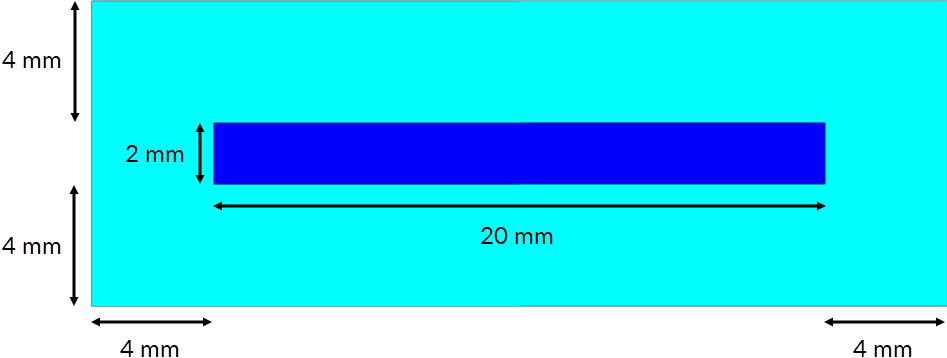Remember, our model will be axisymmetric around the Y axis. As such, what we'll create in Designer will actually be half of the above and will look as so: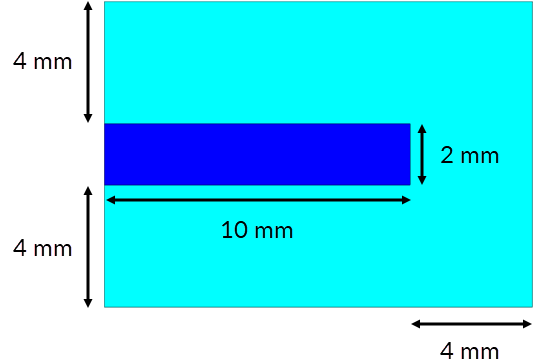We've included the dimensions here. We could just go ahead and add the primitives we'll need, entering these dimensions directly. Instead, however, let's add some parameters! Using parameters makes it easy to apply changes to your model. Also, by using a "varying" parameter, you can sweep different values when running simulations, to see how changes in a parameter's value impact the results.

Here's the model again, but with parameter names in place of dimensions: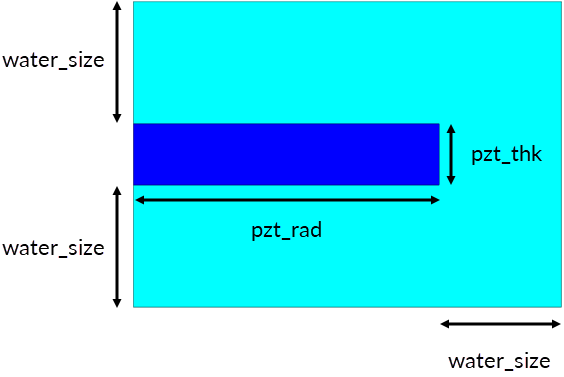We'll also need a few extra parameters to define the start and end points of the PZT on the Y-axis, and the end points of the water: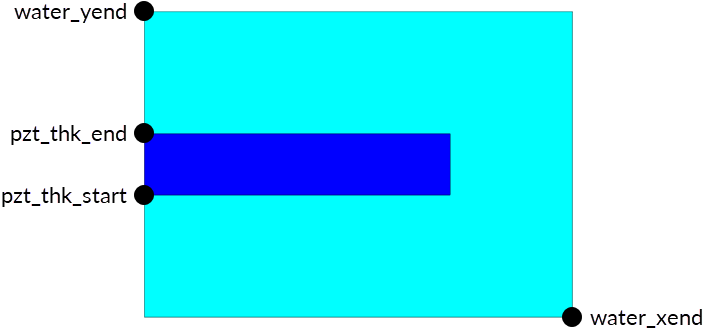## The Parameter Table

You manage parameters using the Parameter Table, and you can add new parameters at the bottom of this window.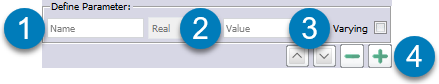1. Type a name for the parameter. This cannot include spaces (but you can use underscores).
2. Type a value for the parameter. You can type a calculation: just start with =. For more about calculations, see Parameter Table.
3. If you want to be able to sweep different values for this parameter when running a simulation, select the Varying checkbox.
4. Click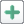.

Now that you know how to add parameters, add the following parameters.

Name Value Varying
water_size 4e-3  Yes
pzt_thick 2e-3 Yes
pzt_thk_start  4e-3  -
pzt_thk_end  =pzt_thk_start + pzt_thick  -